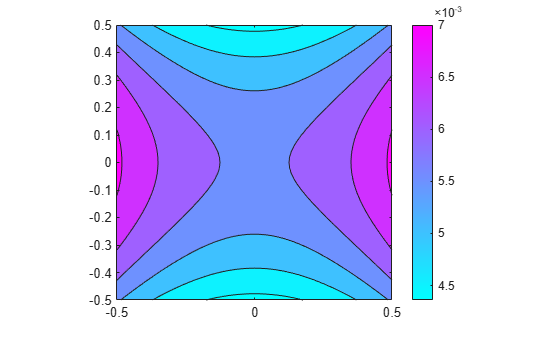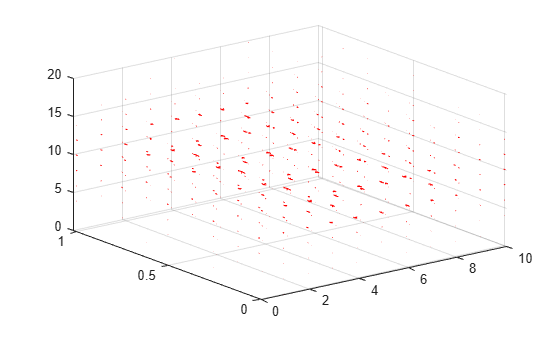interpolateMagneticPotential

Interpolate magnetic potential in magnetostatic result at arbitrary spatial locations

Description

example

Aintrp = interpolateMagneticPotential(magnetostaticresults,xq,yq) returns the interpolated magnetic potential values at the 2-D points specified in xq and yq. .

example

Aintrp = interpolateMagneticPotential(magnetostaticresults,xq,yq,zq) uses 3-D points specified in xq, yq, and zq.

example

Aintrp = interpolateMagneticPotential(magnetostaticresults,querypoints) returns the interpolated magnetic potential values at the points specified in querypoints.

Examples

collapse all

Create an electromagnetic model for magnetostatic analysis.

emagmodel = createpde('electromagnetic','magnetostatic');

Create a square geometry and include it in the model. Plot the geometry with the edge labels.

R1 = [3,4,-1,1,1,-1,1,1,-1,-1]';
g = decsg(R1,'R1',('R1')');
geometryFromEdges(emagmodel,g);
pdegplot(emagmodel,'EdgeLabels','on')
xlim([-1.5 1.5])
axis equalSpecify the vacuum permeability in the SI system of units.

emagmodel.VacuumPermeability = 1.2566370614E-6;

Specify the relative permeability of the material.

electromagneticProperties(emagmodel,'RelativePermeability',5000);

Apply the magnetic potential boundary conditions on the boundaries of the square.

electromagneticBC(emagmodel,'MagneticPotential',0,'Edge',[1 3]);
electromagneticBC(emagmodel,'MagneticPotential',0.01,'Edge',[2 4]);

Specify the current density for the entire geometry.

electromagneticSource(emagmodel,'CurrentDensity',0.5);

Generate the mesh.

generateMesh(emagmodel);

Solve the model and plot the magnetic potential.

R = solve(emagmodel);
pdeplot(emagmodel,'XYData',R.MagneticPotential, ...
'Contour','on')
axis equalInterpolate the resulting magnetic potential to a grid covering the central portion of the geometry, for x and y from -0.5 to 0.5.

v = linspace(-0.5,0.5,51);
[X,Y] = meshgrid(v);
Aintrp = interpolateMagneticPotential(R,X,Y)
Aintrp = 2601×1

0.0056
0.0057
0.0058
0.0059
0.0060
0.0061
0.0062
0.0063
0.0064
0.0065
⋮

Reshape Aintrp and plot the resulting magnetic potential.

Aintrp = reshape(Aintrp,size(X));
figure
contourf(X,Y,Aintrp)
colormap(cool)
colorbarAlternatively, you can specify the grid by using a matrix of query points.

querypoints = [X(:),Y(:)]';
Aintrp = interpolateMagneticPotential(R,querypoints);

Create an electromagnetic model for magnetostatic analysis.

emagmodel = createpde('electromagnetic','magnetostatic');

Import and plot the geometry representing a plate with a hole.

importGeometry(emagmodel,'PlateHoleSolid.stl');
pdegplot(emagmodel,'FaceLabels','on','FaceAlpha',0.3)Specify the vacuum permeability value in the SI system of units.

emagmodel.VacuumPermeability = 1.2566370614E-6;

Specify the relative permeability of the material.

electromagneticProperties(emagmodel,'RelativePermeability',5000);

Specify the current density for the entire geometry.

electromagneticSource(emagmodel,'CurrentDensity',[0;0;0.5]);

Apply the magnetic potential boundary conditions on the side faces and the face bordering the hole.

electromagneticBC(emagmodel,'MagneticPotential',[0;0;0],'Face',3:6);
electromagneticBC(emagmodel,'MagneticPotential',[0;0;0.01],'Face',7);

Generate the linear mesh.

generateMesh(emagmodel);

Solve the model.

R = solve(emagmodel)
R =
MagnetostaticResults with properties:

MagneticPotential: [1×1 FEStruct]
MagneticField: [1×1 FEStruct]
MagneticFluxDensity: [1×1 FEStruct]
Mesh: [1×1 FEMesh]

Plot the magnetic potential.

pdeplot3D(emagmodel,'FlowData',[R.MagneticPotential.Ax ...
R.MagneticPotential.Ay ...
R.MagneticPotential.Az])Interpolate the resulting magnetic potential to a grid covering the entire geometry, for x, y, and z.

x = linspace(0,10,11);
y = linspace(0,1,5);
z = linspace(0,20,11);
[X,Y,Z] = meshgrid(x,y,z);
Aintrp = interpolateMagneticPotential(R,X,Y,Z)
Aintrp =
FEStruct with properties:

Ax: [605×1 double]
Ay: [605×1 double]
Az: [605×1 double]

Reshape Aintrp.Ax, Aintrp.Ay, and Aintrp.Az to match the shape of the input grid.

AintrpX = reshape(Aintrp.Ax,size(X));
AintrpY = reshape(Aintrp.Ay,size(Y));
AintrpZ = reshape(Aintrp.Az,size(Z));

Plot the resulting magnetic potential.

figure
quiver3(X,Y,Z,AintrpX,AintrpY,AintrpZ,'Color','red')Input Arguments

collapse all

Solution of a magnetostatic problem, specified as a MagnetostaticResults object. Create magnetostaticresults using the solve function.

Example: magnetostaticresults = solve(emagmodel)

x-coordinate query points, specified as a real array. interpolateMagneticPotential evaluates the magnetic potential at the 2-D coordinate points [xq(i) yq(i)] or at the 3-D coordinate points [xq(i) yq(i) zq(i)] for every i. Because of this, xq, yq, and (if present) zq must have the same number of entries.

interpolateMagneticPotential converts query points to column vectors xq(:), yq(:), and (if present) zq(:). It returns magnetic potential values as a column vector of the same size. To ensure that the dimensions of the returned solution are consistent with the dimensions of the original query points, use reshape. For example, use Aintrp = reshape(Aintrp,size(xq)).

Example: xq = [0.5 0.5 0.75 0.75]

Data Types: double

y-coordinate query points, specified as a real array. interpolateMagneticPotential evaluates the magnetic potential at the coordinate points [xq(i),yq(i)] for every i. Because of this, xq and yq must have the same number of entries.

interpolateMagneticPotential converts query points to column vectors xq(:), yq(:), and (if present) zq(:). It returns magnetic potential values as a column vector of the same size. To ensure that the dimensions of the returned solution are consistent with the dimensions of the original query points, use reshape. For example, use Aintrp = reshape(Aintrp,size(yq)).

Example: yq = [1 2 0 0.5]

Data Types: double

z-coordinate query points, specified as a real array. interpolateMagneticPotential evaluates the magnetic potential at the 3-D coordinate points [xq(i) yq(i) zq(i)]. Therefore, xq, yq, and zq must have the same number of entries.

interpolateMagneticPotential converts the query points to column vectors xq(:), yq(:), and zq(:). It returns magnetic potential values as a column vector of the same size. To ensure that the dimensions of the returned solution are consistent with the dimensions of the original query points, use reshape. For example, use Aintrp = reshape(Aintrp,size(zq)).

Example: zq = [1 1 0 1.5]

Data Types: double

Query points, specified as a real matrix with either two rows for 2-D geometry or three rows for 3-D geometry. interpolateMagneticPotential evaluates the magnetic potential at the coordinate points querypoints(:,i) for every i, so each column of querypoints contains exactly one 2-D or 3-D query point.

Example: For a 2-D geometry, querypoints = [0.5 0.5 0.75 0.75; 1 2 0 0.5]

Data Types: double

Output Arguments

collapse all

Magnetic potential at query points, returned as a vector for a 2-D problem or an FEStruct object for a 3-D problem. The properties of FEStruct contain the components of the magnetic potential at query points. For query points i that are outside the geometry, Aintrp(i), Aintrp.Ax(i), Aintrp.Ay(i), and Aintrp.Az(i) are NaN. Properties of an FEStruct object are read-only.